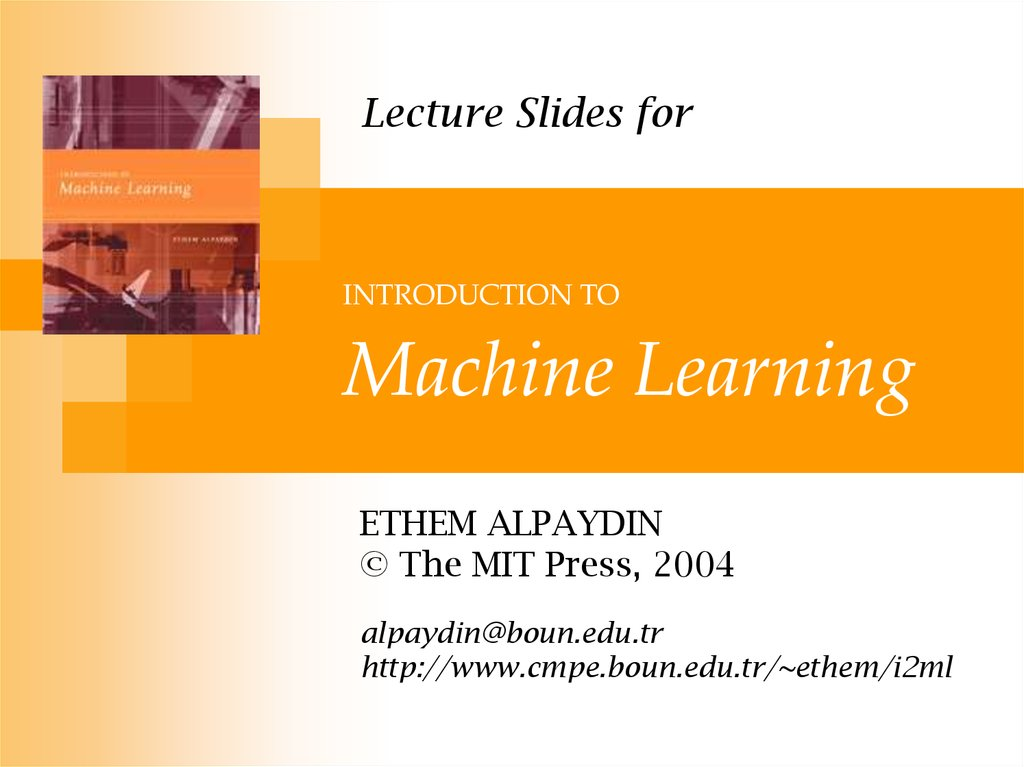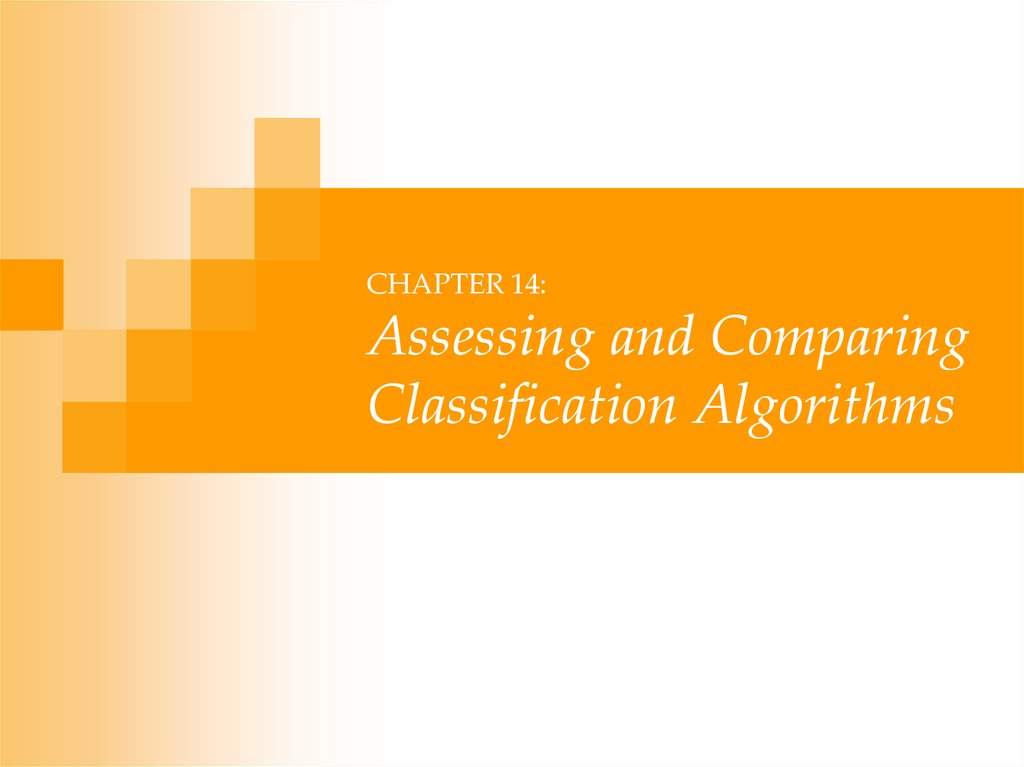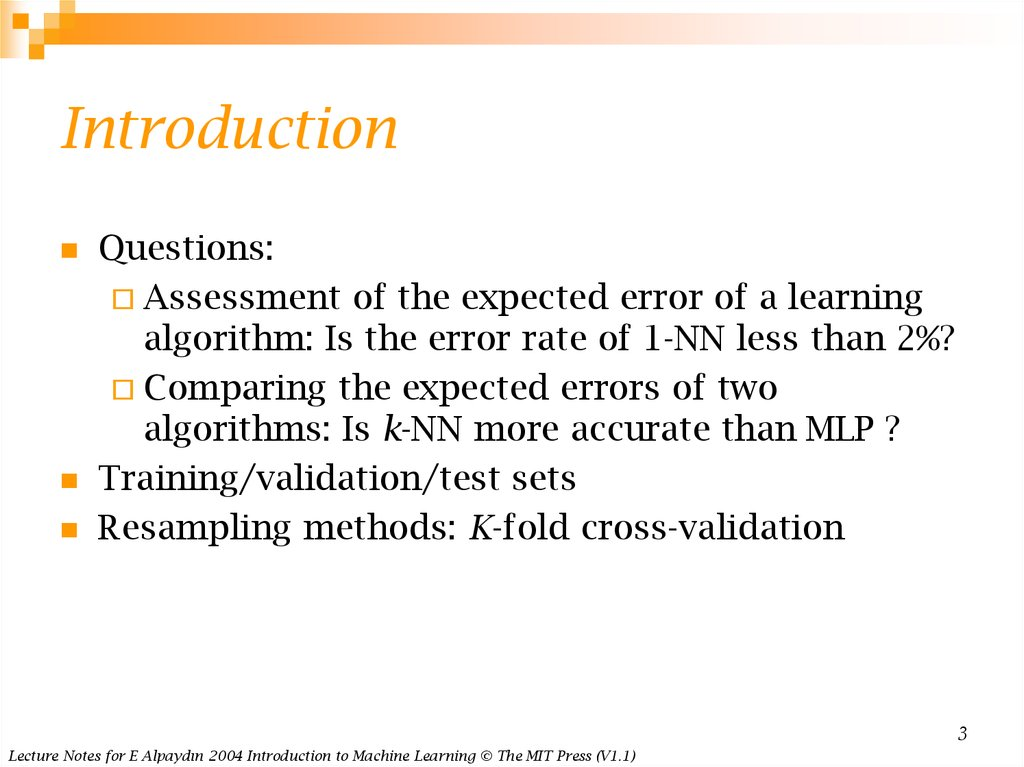# Assessing and comparing classification algorithms

## 1. INTRODUCTION TO Machine Learning

Lecture Slides for
INTRODUCTION TO
Machine Learning
ETHEM ALPAYDIN
[email protected]
http://www.cmpe.boun.edu.tr/~ethem/i2ml

## 2.

CHAPTER 14:
Assessing and Comparing
Classification Algorithms

## 3. Introduction

Questions:
Assessment of the expected error of a learning
algorithm: Is the error rate of 1-NN less than 2%?
Comparing the expected errors of two
algorithms: Is k-NN more accurate than MLP ?
Training/validation/test sets
Resampling methods: K-fold cross-validation
3
Lecture Notes for E Alpaydın 2004 Introduction to Machine Learning © The MIT Press (V1.1)

## 4. Algorithm Preference

Criteria (Application-dependent):
Misclassification error, or risk (loss functions)
Training time/space complexity
Testing time/space complexity
Interpretability
Easy programmability
Cost-sensitive learning
4
Lecture Notes for E Alpaydın 2004 Introduction to Machine Learning © The MIT Press (V1.1)

## 5. Resampling and K-Fold Cross-Validation

The need for multiple training/validation sets
{Xi,Vi}i: Training/validation sets of fold i
K-fold cross-validation: Divide X into k, Xi,i=1,...,K
V1 X1
T 1 X2 X3 X K
V2 X2
T 2 X1 X 3 X K
VK X K T K X1 X2 X K 1
Ti share K-2 parts
5
Lecture Notes for E Alpaydın 2004 Introduction to Machine Learning © The MIT Press (V1.1)

## 6. 5×2 Cross-Validation

5 times 2 fold cross-validation (Dietterich, 1998)
T 1 X1 1
V1 X1 2
2
1
T 2 X1
V2 X1
T 3 X2 1
V3 X2 2
T 4 X2 2
V4 X2 1
T 9 X5 1
2
T 10 X5
V9 X5 2
1
V10 X5
6
Lecture Notes for E Alpaydın 2004 Introduction to Machine Learning © The MIT Press (V1.1)

## 7. Bootstrapping

Draw instances from a dataset with replacement
Prob that we do not pick an instance after N draws
N
1
1
1 e 0.368
N
that is, only 36.8% is new!
7
Lecture Notes for E Alpaydın 2004 Introduction to Machine Learning © The MIT Press (V1.1)

## 8. Measuring Error

Error rate
Recall
= # of errors / # of instances = (FN+FP) / N
= # of found positives / # of positives
= TP / (TP+FN) = sensitivity = hit rate
Precision = # of found positives / # of found
= TP / (TP+FP)
Specificity = TN / (TN+FP)
False alarm rate = FP / (FP+TN) = 1 - Specificity
8
Lecture Notes for E Alpaydın 2004 Introduction to Machine Learning © The MIT Press (V1.1)

## 9. ROC Curve

9
Lecture Notes for E Alpaydın 2004 Introduction to Machine Learning © The MIT Press (V1.1)

## 10. Interval Estimation

X = { xt }t where xt ~ N ( μ, σ2)
m ~ N ( μ, σ2/N)
N
m ~ Z
m
P 1.96 N
1.96 0.95
P m 1.96
m 1.96
0.95
N
N
P m z / 2
m z / 2
1
N
N
Lecture Notes for E Alpaydın 2004 Introduction to Machine Learning © The MIT Press (V1.1)
100(1- α) percent
confidence
interval
10

## 11.

m
P N
1.64 0.95
P m 1.64
0.95
N
P m z
1
N
When
σ2 is not known:
S x m / N 1
2
t
t
2
N m
~ t N 1
S
S
S
P m t / 2,N 1
m t / 2,N 1
1
N
N
11
Lecture Notes for E Alpaydın 2004 Introduction to Machine Learning © The MIT Press (V1.1)

## 12. Hypothesis Testing

Reject a null hypothesis if not supported by the
sample with enough confidence
X = { xt }t where xt ~ N ( μ, σ2)
H0: μ = μ0 vs. H1: μ ≠ μ0
Accept H0 with level of significance α if μ0 is in the
100(1- α) confidence interval
N m 0
z / 2 , z / 2
Two-sided test
12
Lecture Notes for E Alpaydın 2004 Introduction to Machine Learning © The MIT Press (V1.1)

## 13.

One-sided test: H0: μ ≤ μ0 vs. H1: μ > μ0
Accept if
N m 0
, z
Variance unknown: Use t, instead of z
Accept H0: μ = μ0 if
N m 0
t / 2,N 1 ,t / 2,N 1
S
13
Lecture Notes for E Alpaydın 2004 Introduction to Machine Learning © The MIT Press (V1.1)

## 14. Assessing Error: H0: p ≤ p0 vs. H1: p > p0

Assessing Error:
H0: p ≤ p0 vs. H1: p > p0
Single training/validation set: Binomial Test
If error prob is p0, prob that there are e errors or
less in N validation trials is
N j
j
P X e p 0 1 p 0
j 1 j
e
N j
Accept if this prob is less than 1- α
1- α
N=100, e=20
Lecture Notes for E Alpaydın 2004 Introduction to Machine Learning © The MIT Press (V1.1)
14

## 15. Normal Approximation to the Binomial

Number of errors X is approx N with mean Np0 and
var Np0(1-p0)
X Np 0
~Z
Np 0 1 p 0
Accept if this prob for X = e is
less than z1-α
1- α
15
Lecture Notes for E Alpaydın 2004 Introduction to Machine Learning © The MIT Press (V1.1)

## 16. Paired t Test

Multiple training/validation sets
xti = 1 if instance t misclassified on fold i
N
t
Error rate of fold i:
x
t 1 i
pi
N
2
With m and s average and var of pi
we accept p0 or less error if
K m p 0
~ t K 1
S
is less than tα,K-1
16
Lecture Notes for E Alpaydın 2004 Introduction to Machine Learning © The MIT Press (V1.1)

## 17. Comparing Classifiers: H0: μ0 = μ1 vs. H1: μ0 ≠ μ1

Single training/validation set: McNemar’s Test
Under H0, we expect e01= e10=(e01+ e10)/2
e
e10 1
2
01
e01 e10
~ X12
Accept if < X2α,1
17
Lecture Notes for E Alpaydın 2004 Introduction to Machine Learning © The MIT Press (V1.1)

## 18. K-Fold CV Paired t Test

Use K-fold cv to get K training/validation folds
pi1, pi2: Errors of classifiers 1 and 2 on fold i
pi = pi1 – pi2 : Paired difference on fold i
The null hypothesis is whether pi has mean 0
H 0 : 0 vs. H 0 : 0
i 1pi
K
m
K
K m 0
s
2
p
m
i 1 i
K
s2
K 1
K m
~ t K 1 Accept if in t / 2,K 1 ,t / 2,K 1
s
18
Lecture Notes for E Alpaydın 2004 Introduction to Machine Learning © The MIT Press (V1.1)

## 19. 5×2 cv Paired t Test

Use 5×2 cv to get 2 folds of 5 tra/val replications
(Dietterich, 1998)
pi(j) : difference btw errors of 1 and 2 on fold j=1, 2
of replication i=1,...,5
1
2
pi pi pi
/ 2
2
i
1
s pi pi
p1 1
2
s
i 1 i / 5
5
p
2
2
i
pi
2
~ t5
Two-sided test: Accept H0: μ0 = μ1 if in (-tα/2,5,tα/2,5)
One-sided test: Accept H0: μ0 ≤ μ1 if < tα,5
19
Lecture Notes for E Alpaydın 2004 Introduction to Machine Learning © The MIT Press (V1.1)

## 20. 5×2 cv Paired F Test

p
2 s
j
5
2
i 1
i
j 1
5
2
i 1 i
2
~ F10 ,5
Two-sided test: Accept H0: μ0 = μ1 if < Fα,10,5
20
Lecture Notes for E Alpaydın 2004 Introduction to Machine Learning © The MIT Press (V1.1)

## 21. Comparing L>2 Algorithms: Analysis of Variance (Anova)

Comparing L>2 Algorithms:
Analysis of Variance (Anova)
H 0 : 1 2 L
Errors of L algorithms on K folds
X ij ~ N j , 2 , j 1,..., L, i 1,..., K
We construct two estimators to σ2 .
One is valid if H0 is true, the other is always valid.
We reject H0 if the two estimators disagree.
21
Lecture Notes for E Alpaydın 2004 Introduction to Machine Learning © The MIT Press (V1.1)

## 22.

If H 0 is true :
K
mj
i 1
X ij
~ N , 2 / K
K
j 1m j
L
m
S
2
m
j
m
2
j
L
L 1
Thus an estimator of 2 is K S 2 , namely,
L
ˆ 2 K
j
m
m
j 1
m
/K
2
j
L 1
2
j
2
m
~ X L2 1 SSb K m j m
2
j
So when H 0 is true, we have
SSb
2
~
X
L 1
2
22
Lecture Notes for E Alpaydın 2004 Introduction to Machine Learning © The MIT Press (V1.1)

## 23.

Regardless of H 0 our second estimator to 2 is the
average of group variances S 2j :
X
K
S 2j
i 1
mj
2
ij
L
2
ˆ
K 1
SSw X ij m j
j 1
S 2j
L
j
i
X
mj
2
ij
L K 1
2
j
K 1
S 2j
i
~X
2
K 1
SSw
2
2
SSb / 2 SSw / 2
~ X L2 K 1
SSb / L 1
/
~ FL 1,L K 1
L 1 L K 1 SSw / L K 1
H 0 : 1 2 L if F ,L 1,L K 1
23
Lecture Notes for E Alpaydın 2004 Introduction to Machine Learning © The MIT Press (V1.1)

## 24. Other Tests

Range test (Newman-Keuls): 145 23
Nonparametric tests (Sign test, Kruskal-Wallis)
Contrasts: Check if 1 and 2 differ from 3,4, and 5
Multiple comparisons require Bonferroni correction
If there are m tests, to have an overall significance
of α, each test should have a significance of α/m.
Regression: CLT states that the sum of iid variables
from any distribution is approximately normal and
the preceding methods can be used.
Other loss functions ?
24
Lecture Notes for E Alpaydın 2004 Introduction to Machine Learning © The MIT Press (V1.1)